# Solving an Asymmetrical Inequalities Problem: Seeking Light

Homework Statement
If x1+x2+x3...+xn=0 and x1^2+x2^2...+xn^2=1, with x1,x2,x3...,xn ∈ ℝ. What is the maximum value of x1^3+x2^3+...+xn^3?
Relevant Equations
I couldn't think of a way to solve this with MA greater than MG, but apparently I should use this, any other way is welcome
I'm obsessed with this problem and need some light, I've tried solving it using inequalities but there seems to be an asymmetry that's hard to deal with.

Welcome to PF!

Can you provide some context for your problem? is this from a math olympiad? where did you see this problem?

Can you show us what you tried?

What is MA and what is MG?

Right off the bat one asymmetry would be that the first equation in order to be zero must have some positive and negative ##x_i## numbers whereas the second one squares the ##x_i## numbers so they must all be positive.

Have you tried to see what if its just one number, then just two numbers, ...? to see if there's a trend?

•Homework Statement:: If x1+x2+x3...+xn=0 and x1^2+x2^2...+xn^2=1, with x1,x2,x3...,xn ∈ ℝ. What is the maximum value of x1^3+x2^3+...+xn^3?
Relevant Equations:: I couldn't think of a way to solve this with MA greater than MG, but apparently I should use this, any other way is welcome

I'm obsessed with this problem and need some light, I've tried solving it using inequalities but there seems to be an asymmetry that's hard to deal with.
Have you checked for ##n=1## and ##n=2## to see where it leads you to?
Then ##n=3## probably behaves like the general case.

The general case is an ellipsoid ##\displaystyle{\sum_{k=1}^n x_k^2=1}## intersected by the plane ##\displaystyle{\sum_{k=1}^n x_k=0}.## This is a conic section of dimension ##n-1.## I assume an ellipsoid. Finally, we want to maximize the function ##f(x)=\displaystyle{\sum_{k=1}^n x_k^3}## on that ellipsoid. Looks, as if it could be done numerically, or by standard methods of analysis.

You can find useful techniques and theorems at

July 2021: Heron's theorem
January 2020: MacLaurin's identity, Newton's identity
December 2019: Nesbitt's identity, Mahler's identity

•jedishrfu, Luckyroad21, MatinSAR and 1 other person
Welcome to PF!

Can you provide some context for your problem? is this from a math olympiad? where did you see this problem?

Can you show us what you tried?

What is MA and what is MG?

Right off the bat one asymmetry would be that the first equation in order to be zero must have some positive and negative ##x_i## numbers whereas the second one squares the ##x_i## numbers so they must all be positive.

Have you tried to see what if its just one number, then just two numbers, ...? to see if there's a trend?
I was challenged by a friend, MA would be the arithmetic mean and MG would be the geometric mean. So, through the analysis of the initial cases I conjectured that the solution would be something like x1=x2=x3=...=xn-1= -(n^2+n)^(-1/2) and xn= (1- 1/n)^(1/2), this conjecture is right for n=2 (trivial case) and n=3 (I analyzed the graph of the third degree polynomial that appears as a function of one of the variables when isolating it). Forgive the English, I'm very dumb and that's not my language.

To solve the elegant asymmetry, I took ai = 1/n + xi. (n^2-n)^(-1/2), such a transformation led me to non-negative real numbers such that:
a1+a2+a3...+an=1
It is
a1^2+a2^2+a3^2...+an^2= (n-1)^(-1). The problem is to get the maximum value of a1^3+a2^3+a3^3...+an^3.

\begin{align*}
1&=\sum_{k=1}^n x_k^2 = x_1^2+\sum_{k=2}^n x_k^2=\left(-\sum_{k=2}^n x_k\right)^2 +\sum_{k=2}^n x_k^2 = \sum_{i,j=2}^n x_i x_j +\sum_{k=2}^n x_k^2\\
&=\sum_{2\leq i<j\leq n}2x_i x_j+2\sum_{k=2}^n x_k^2 = 2 \sum_{2\leq i\leq j\leq n}x_i x_j
\end{align*}
Now set
$$\Lambda (\lambda,x_1,x_2,\ldots,x_n ):= \sum_{k=1}^n x_k^3 +\lambda \cdot \left( -\dfrac{1}{2}+\sum_{2\leq i\leq j\leq n}x_i x_j \right)$$
and find ##\nabla \Lambda = 0.##

https://en.wikipedia.org/wiki/Lagrange_multiplier

•This seems like a standard Lagrange multipliers problem.

•I applied the partial derivatives you suggested and arrived at x1=0, the problem is that for n=3, the optimal solution does not contain null terms. I apologize if this is too obvious, I'm just a humble medical student who likes numbers.

I applied the partial derivatives you suggested and arrived at x1=0, the problem is that for n=3, the optimal solution does not contain null terms. I apologize if this is too obvious, I'm just a humble medical student who likes numbers.
I haven't done the exercise. You could e.g. assume w.l.o.g. ##x_1 \geq x_2 \geq x_3 \geq \ldots \geq x_n.##
Then ##x_1=-\sum_{k=2}^n x_k\,## and
\begin{align*}
1-x_1^2&=(1-x_1)(1+x_1)=\sum_{k=2}^n x_k^2=\left(1+\sum_{k=2}^n x_k\right)\left(1-\sum_{k=2}^n x_k\right)\\[6pt]
&=1-\left(\sum_{k=2}^n x_k\right)^2=1-\sum_{k=2}^n x_k^2-2\sum_{2\leq i < j\leq n}x_i x_j\\
\dfrac{1}{2}&= \sum_{2\leq i \leq j\leq n}x_i x_j =\sum_{k=2}^n x_k^2+ \sum_{2\leq i < j\leq n}x_i x_j=1-x_1^2+ \sum_{2\leq i < j\leq n}x_i x_j\\[6pt]
0&=\dfrac{1}{2}-x_1^2+ \sum_{2\leq i < j\leq n}x_i x_j
\end{align*}
$$\Lambda(\lambda ,x_1,\ldots,x_n)=\sum_{k=1}^n x_k^3 +\lambda \left(\dfrac{1}{2}-x_1^2+ \sum_{2\leq i < j\leq n}x_i x_j\right)$$
\begin{align*}
\dfrac{\partial \Lambda}{\partial \lambda }&=\dfrac{1}{2}-x_1^2+ \sum_{2\leq i < j\leq n}x_i x_j\\[6pt]
\dfrac{\partial \Lambda}{\partial x_1}&=3x_1^2-2\lambda x_1\\[6pt]
\dfrac{\partial \Lambda}{\partial x_k}&=3x_k^2+\lambda \sum_{j=2,j\neq k}^n x_j \, , \,k>1
\end{align*}
etc.

This way, we don't eliminate a variable.

•##n=2## has to be solved manually since the feasible set is a single point.

My calculations for ##n=3## had the solution ##\sqrt{1/6}## at
$$(x_1\, , \,x_2\, , \,x_3)=\left(\sqrt{\dfrac{2}{3}}\, , \,-\sqrt{\dfrac{1}{6}}\, , \,-\sqrt{\dfrac{1}{6}}\right)$$
but my calculations are one giant scribbling, so they could be wrong.

Your result for n=3 is positive for my conjecture.For n=4, the solutions I obtained from the partial derivatives do not follow the imposed restrictions. For forcing such restrictions on the obtained system I arrived at xi=(2/n)^(1/2) or xi= - (1/2n)^(1/2); it's easy to see that this only adds up to zero if n is a multiple of 3

Your result for n=3 is positive for my conjecture.For n=4, the solutions I obtained from the partial derivatives do not follow the imposed restrictions. For forcing such restrictions on the obtained system I arrived at xi=(2/n)^(1/2) or xi= - (1/2n)^(1/2); it's easy to see that this only adds up to zero if n is a multiple of 3
My crucial conditions are
$$\dfrac{1}{2}=x_1^2- \sum_{2\leq i < j \leq n} x_ix_j \quad\text{and}\quad x_k\in \left\{x_1\, , \,-\dfrac{x_1}{2}\right\}\quad\text{and}\quad x_1+x_2+\ldots+x_n=0$$
under the assumption ##x_1\geq x_2 \geq \ldots\geq x_n## and that I didn't make a mistake, especially no sign error anywhere.

Let ##x_k=x_1 \varepsilon_k## with ##\varepsilon_k\in \{1,-1/2\}## and ##\underbrace{x_1 = \ldots = x_1}_{p\text{ times}} >0>\underbrace{-\frac{x_1}{2}=\ldots=-\frac{x_1}{2}}_{q\text{ times}}## and ##p+q=n## these read as
\begin{align*}
\end{align*}
with ##1=\varepsilon_1=\varepsilon_2=\ldots=\varepsilon_{p}## and ##-1/2=\varepsilon_{p+1}=\ldots=\varepsilon_n.##

We get for ##n=3## that ##(p,q)\in \{(0,3),(1,2),(2,1),(3,0)\}## where only ##(p,q)=(1,2)## fulfills ##2p=q.## Hence
$$\dfrac{1}{2}=x_1^2\left(1-\varepsilon_2\varepsilon_3\right)=x_1^2\cdot \dfrac{3}{4}\text{ and }x_1=\sqrt{\dfrac{2}{3}}\, , \,x_2=x_3=-\sqrt{\dfrac{1}{6}}.$$

So all depends on whether (*) has a solution, i.e. whether ##3\,|\,n.##

Last edited:
•However, the set of feasible points
$$\mathcal{F}_n:=\left\{(x_1,\ldots,x_n)\in \mathbb{R}^n\,|\,\sum_{k=1}^nx_k=0\; \wedge \sum_{k=1}^nx_k^2=1\;\right\}$$
should be compact. So ##\mathcal{F}_n=\emptyset## or the optimization problem has a solution.

•So what do you mean is that we only retrieve the solutions for n multiple 3? Do the others not exist?

To solve the elegant asymmetry, I took ai = 1/n + xi. (n^2-n)^(-1/2), such a transformation led me to non-negative real numbers such that:
a1+a2+a3...+an=1
It is
a1^2+a2^2+a3^2...+an^2= (n-1)^(-1). The problem is to get the maximum value of a1^3+a2^3+a3^3...+an^3.
Maybe it's easier to work with that.

##\mathcal{F}_4\neq \emptyset## because ##(1/\sqrt{2},0,0,-1/\sqrt{2})\in \mathcal{F}_4.## We can shuffle the coordinates and get twelve points. All of them have ##\sum x_k^3=0.## I don't know if there are more points. It could be the case that ##\mathcal{F}_n=\{\vec{v}_1,\ldots,\vec{v}_m(n)\}## is a set of finitely many points whenever ##3\, \nmid \,n.##

The method with the Lagrange multiplier doesn't work on sets that consists of isolated points only. That's why e.g. ##n=2## had to be solved manually.

•With :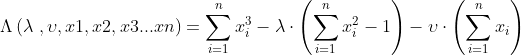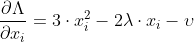(1)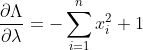(2)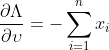(3)

Equating the partial derivatives to 0, we have: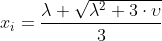or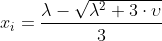(4)

So I will assume that lambda is negative and that there are b numbers of xi's that are negative and (n-b) numbers of xi's that are positive. (5)
Applying (5) and (4) to (3), we get: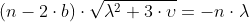(6)
Applying (5) and (4) to (2), we get: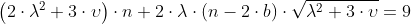(7)

Applying (6) to (7), we get: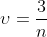(8)

Applying (8) to (6), we get: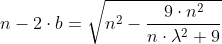(10)

Appyling (6) in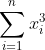, we get: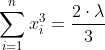So, the smallest value of this sum occurs when lambda is minimum.
Analyzing (10), it is clear that the lambda modulus is maximum when b is minimum, as b is different from 0, we make b =1.
For b=1, with lambda as negative number: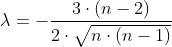Applying this to (4) and choosing x1 to be the negative number, we have: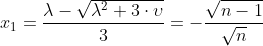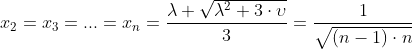Obviously, the set of points that maximizes our sum is equal to the one that minimizes with the signs of the numbers swapped (our sum is an odd function), so: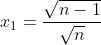and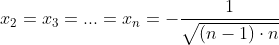Am I right?

Am I right?
I'm not sure. I would like to see the feasible sets first. If some of them are finite and others infinite, the solution has to respect this. It looked to me as if only dimensions divisible by three had infinite feasible sets, however, I'm not sure. Lagrange multipliers require infinite sets on which differentiation can be performed. Thus you have to calculate the feasible sets before you differentiate.

•How can I check this?

How can I check this?
You have to solve the equations: ##x_1+\ldots+x_n=0## and ##x_1^2+\ldots+x_n^2=1.##

•For any Numbers x1,x2…xn with x1+x2…+xn=0 and x1^2*x2^2…+xn^2= u, get xi/sqrt(u)=xi’. We Will have: Sum(xi’)=0 and Sum(xi’^2)=1

This proves that there are infinite solutions

This proves that there are infinite solutions
I set ##n=4## and put it in WA. It gave me only two real solutions:
https://www.wolframalpha.com/input?i=x+y+z+w=0+AND+x^2+y^2+z^2+w^2=1

However, it matches one half of your solution. With ##(x_k)## there is also ##-(x_k)## a solution, so you must check both to decide whether ##\sum x_k^3## or ##-\sum x_k^3## is bigger.

Last edited:
•Using the method, it's easy to find all the other solutions, for example: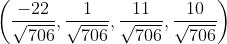Here is an example of building infinite solutions; with m being a positive number: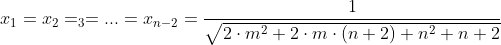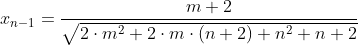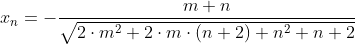Is this enough to be able to apply the Lagrangian method?

Yes.

We need that the target function is differentiable on the feasible set. That's why we need a reasonable set and not only distributed single points (like for ##n=2##) where the derivatives wouldn't exist.

••